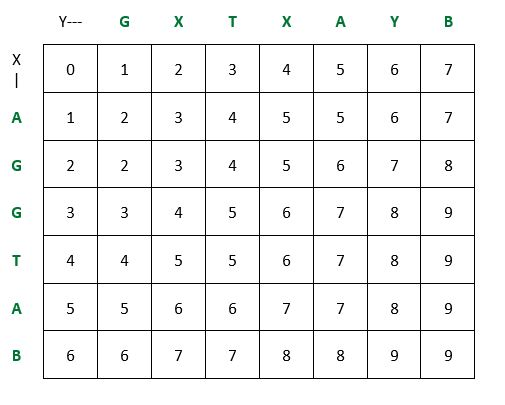# Shortest Common Supersequence Problem Basic Information

suggest change

The Shortest Common Super Sequence is a problem closely related to the longest common subsequence, which you can use as an external function for this task. The shortest common super sequence problem is a problem closely related to the longest common subsequence problem.

A shortest common supersequence (scs) is a common supersequence of minimal length. In the shortest common supersequence problem, the two sequences `X` and `Y` are given and the task is to find a shortest possible common supersequence of these sequences. In general, an scs is not unique.

Given two sequences `X = <x1,...,xm>` and `Y = <y1,...,yn>`, a sequence `U = <u1,...,uk>` is a common super sequence of `X` and `Y` if `U` is a super sequence of both `X` and `Y`. In other words, a shortest common super sequence of strings `x` and `y` is a shortest string `z` such that both `x` and `y` are subsequences of `z`.

For two input sequences, an scs can be formed from a longest common subsequence (lcs) easily. For example, if `X[1..m]=abcbdab` and `Y[1..n]=bdcaba`, the lcs is `Z[1..r]=bcba`. By inserting the non-lcs symbols while preserving the symbol order, we get the scs: `U[1..t]=abdcabdab`.

It is quite clear that `r+t=m+n` for two input sequences. However, for three or more input sequences this does not hold. Note also, that the lcs and the scs problems are not dual problems.

For the more general problem of finding a string, `S` which is a superstring of a set of strings `S1,S2,...,Sl`, the problem is NP-Complete . Also, good approximations can be found for the average case but not for the worst case.

Example of Shortest Common Supersequence Problem:Time Complexity: `O(max(m,n))`